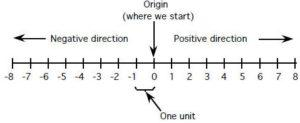# Tag: number line

## Number SystemIn early civilizations, the number of animals (sheep, goat, and camel etc.) or children people have were tracked by using different methods such as people match the number of animals with the number of stones. Similarly, they count the number of children with the number of notches tied on a string or marks on a piece of wood, leather or wall. With the development of human, other uses for numerals were found and this led to the invention of the number system.

## Natural Numbers

Natural numbers are used to count the number of subjects or objects. Natural numbers are also called counting numbers. The numbers$1, 2, 3, \cdots$

are all natural numbers.

## Whole Numbers

The numbers$0, 1, 2, \cdots$ are called whole numbers. It can be observed that whole numbers except 0 are natural numbers.

## Number Line

Whole numbers can be represented by points on a line called the number line. For this purpose, a straight line is drawn and a point is chosen on the line and labeled as 0. Starting with 0, mark off equal intervals of any suitable length. Marked points are labeled as$1, 2, \cdots$ as shown in Figure below. The figure below represents real numbers since it includes the negative number (numbers on the left of 0 in this diagram are called negative numbers).The arrow on the extreme (right-hand side in case of while numbers or negative numbers) indicates that the list of numbers continues in the same way indefinitely.

A whole number can be even or odd. An even number is a number which can be divided by 2 without leaving any remainder. The numbers$0, 2, 4, 6, 8, \cdots$ are all even numbers. An odd number is a number which cannot be divided by 2 without leaving any remainders. The numbers$1, 3, 5, 7, 9, \cdots$ are all odd numbers.

It is interesting to know that any two numbers can be added in any order and it will not affect the results. For example,$3+5 = 5+3$. This is called the commutative law of addition. Similarly, the order of grouping the numbers together does not affect the result. For example,$2+3+5=(2+3)+5 = 2+ (3+5)=(2+5)+3$. This is called the associative law of addition. The subtraction and division of numbers are not commutative as$5-7\ne7-5$ and$6\div2 \ne 2\div 6$ in general.

Like addition and multiplication, whole numbers also follow commutative law and it is called commutative law of multiplication, for example,$2\times 8 = 8 \times 2$. Like addition and multiplication, whole numbers also follow associative law of multiplications. For example,$2 \times (3 \times 4) = (2 \times 3) \times 4 = (2 \times 4)\times 3$. Similarly, multiplication is distributive over addition and subtraction, for example, (i)$5\times (6 + 7) = (5 \time 6) + (5 \times 7)$ or$(6+7) \times 5=(6 \times 5)+(7 \times 5)$. (ii)$3 \times (6-2) = (3 \times 6) - (3 \times 2)$ or$(6-2) \times 3 = (6 \times 3) - (2 \times 3)$.

Take any two digit number say 57, reverse the digits to obtain 75. Now subtract the smaller number from the bigger number, we have$75-57=18$. Now reverse the digits of 18 and add 18 to its reverse (81), that is, 18+81, you will get 99.